×#### Thank you for registering.

One of our academic counsellors will contact you within 1 working day.

Click to Chat

1800-1023-196

+91-120-4616500

CART 0

• 0

MY CART (5)

Use Coupon: CART20 and get 20% off on all online Study Material

ITEM
DETAILS
MRP
DISCOUNT
FINAL PRICE
Total Price: Rs.

There are no items in this cart.
Continue Shopping• Complete JEE Main/Advanced Course and Test Series
• OFFERED PRICE: Rs. 15,900
• View Details

```Chapter 6: Fractions – Exercise 6.9

Question: 1

i) 3/4 and 5/6

ii) 7/10 and 2/15

iii) 8/13 and 2/3

iv) 4/5  and  7/15

Solution:

i) Given: 3/4 and 5/6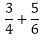LCM of 4 and 6 is 12, so we will convert each fraction into an equivalent fraction with denominator 12.ii) Given: 7/10 and 2/15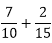LCM of 10 and 15 is 30, so we will convert each fraction into an equivalent fraction with denominator 30.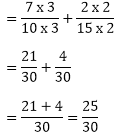iii) Given: 8/13 and 2/3LCM of 13 and 3 is 39, so we will convert each fraction into an equivalent fraction with denominator 39.iv) Given: 4/5 and 7/15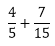LCM of 5 and 15 is 1, so we will convert each fraction into an equivalent fraction with denominator 15.Question: 2

Subtract:Solution:

i) Given: 2/7 and  19/21LCM of 21 and 7 is 21, so we will convert each fraction into an equivalent fraction with denominator 21.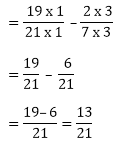ii) Given: 21/25 and 18/20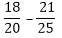LCM of 20 and 25 is 100, so we will convert each fraction into an equivalent fraction with denominator 100.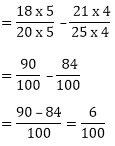iii) Given: 2/1 and 7/16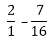LCM of 1 and 16 is 16, so we will convert each fraction into an equivalent fraction with denominator 16.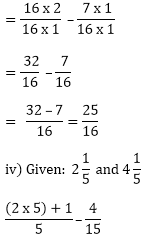LCM of 5 and 15 is 15, so we will convert each fraction into an equivalent fraction with denominator 15.Question: 3

Find the difference of:

i) 13/24 and 7/16

i)  5/18 and 4/15

i)  1/12 and 3/4

i) 2/3 and 6/7

Solution:

i) Given: 13/24 and 7/16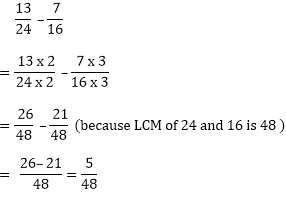ii) Given: 5/18 and 4/15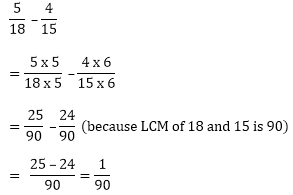iii) Given: 3/4 and 1/12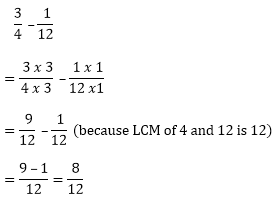iv) Given: 6/7 and 2/3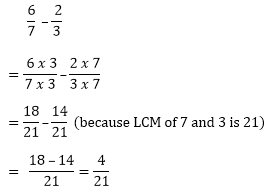Question: 4

Subtract as indicated: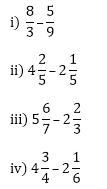Solution:

i) Given: 8/3 and 5/9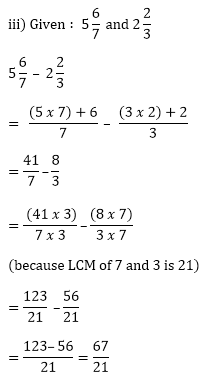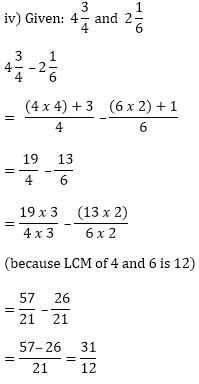Question: 5

Simplify: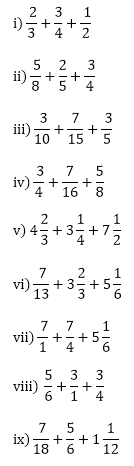Solution: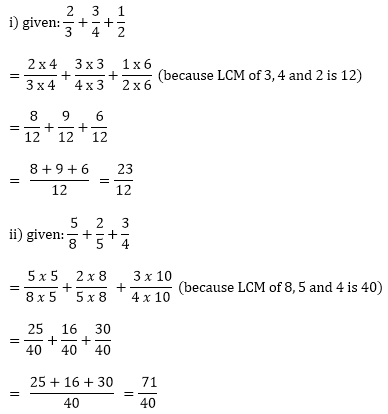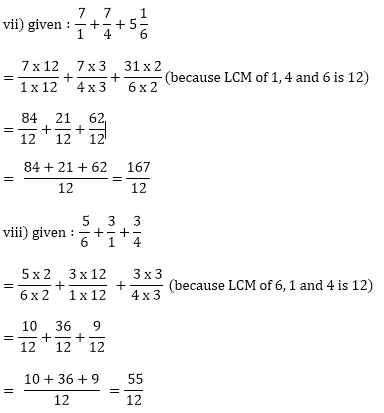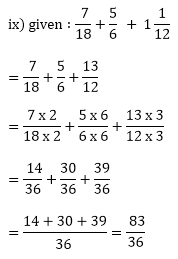Question: 6

Replace * with a correct number: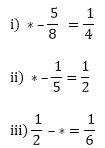Solution: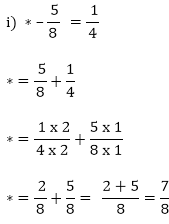Therefore, 7/8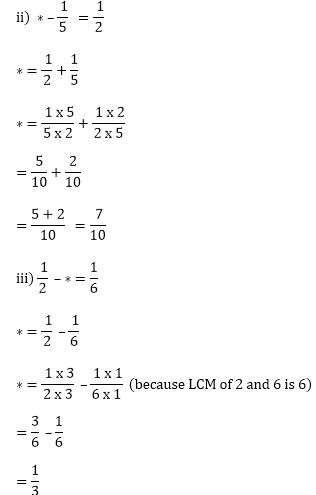Question: 7

Savita bought 2/5 m of ribbon and kavita 3/4 m of ribbon. What was the total length of the ribbon they bought?

Solution:

Length of the ribbon bought by savita = 2/5 metres

Length of the ribbon bought by kavita = 3/4 metres

Total length of the ribbon bought by them: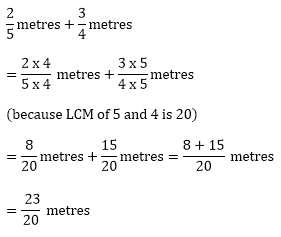Question: 8

Ravish takes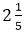minutes to walk across the school ground. Rahul takes 7/4 minutes to do the same. Who takes less time and by what fraction?

Solution:(LCM of 4 and 5 is 20, so will we convert each fraction into an equivalent fraction with denominator 20)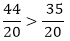Rahul takes less time,Question: 9

A piece of a wire 7/8 metres long broke into two pieces. One piece was 1/4 meter long. How long is the other piece?

Solution:

Length of the wire = 7/8 metres

Length of one piece of wire = 1/4 metres

Let the length of the second piece of wire be x m.

Therefore, Length of the wire = length of one piece + length of the second piece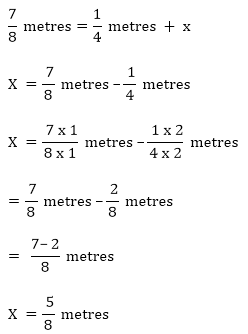Therefore, the length of the second piece is 5/8 metres

Question: 10

Shikha and priya have bookshelves of the same size shikha’s shelf is 5/6 full of book and priya’s shelf is 2/5 full. Whose bookshelf is more full? By what fraction?

Solution:

Fraction of shikha’s filled bookshelf = 5/6

Fraction of Priya’s filled bookshelf = 2/5

Comparing 5/6  and 2/5, we get :

LCM of 5 and 6 is 30, so will convert each fraction into an equivalent fraction with denominator 30.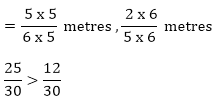Shikha’s shelf is more full.

Therefore,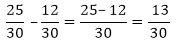Question: 11

Ravish’s house is 9/10 Km from his school. He walked some distance and then took a bus for 1/2 Km. How far did he walk?

Solution:

Total distance between the house and the school = 9/10 Km

Distance covered in the bus = 1/2 Km

Distance covered by walking + distance covered in the bus = total distance between

the house and the school

Distance covered by walking = total distance between the house and the school – Distance

covered in the bus

Distance covered by walking:

9/10 Km – 1/2 Km

LCM of 10 and 2 is 10, so we convert each fraction into an equivalent fraction with denominator 10(HCF of numerator and denominator is 2)
```### Course Features

• 728 Video Lectures
• Revision Notes
• Previous Year Papers
• Mind Map
• Study Planner
• NCERT Solutions
• Discussion Forum
• Test paper with Video Solution